# Linear equation

(diff) ← Older revision | Latest revision (diff) | Newer revision → (diff)

An equation of the form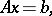(1)

whereis a linear operator acting from a vector spaceinto a vector space,is an unknown element ofandis a given element of(the free term). If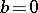, the linear equation is said to be homogeneous. A solution of the linear equation is an elementthat makes (1) an identity: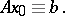The simplest example is a linear operator(a linear function) and the linear algebraic equation determined by it:(2)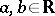or(or an arbitrary field); a solution of it exists if and only if either(and then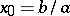) or(and thenis arbitrary). A generalization of equation (2) is a linear equation of the form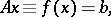(3)

whereis a linear functional defined on a vector spaceover a fieldand. In particular, if the dimension ofis finite and equal to(so thatis isomorphic to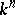), thenis a linear form in several variablesand (3) can be written as(4)

If the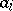are not all zero simultaneously, then the set of solutions of (4) forms an-dimensional linear variety (in the homogeneous case, a linear subspace) in. Ifis infinite dimensional, then the set of solutions of (3) is a linear variety of codimension 1.

Several equations of the form (4) constitute a system of linear equations: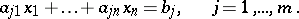(5)

The system (5) can be interpreted as one linear equation of the form (1) if forone takes the spaceand forthe space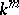, and one specifies the operatorby a matrix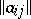,,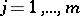. The question of the compatibility of the system of linear equations (5), that is, the question of the existence of solutions of the system of linear equations, is settled by comparing the ranks of the matricesand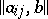: there is a solution if and only if these ranks are equal.

Things are more complicated whenandare infinite-dimensional vector spaces. An important role is played by the topologies of the spacesandand various properties of the operatorsuch as being bounded, continuous, etc., determined by them. In the general case the existence and uniqueness of a solution of a linear equation are determined by the invertibility of(see Inverse mapping). However, it is often far from easy to inverteffectively, and so in the investigation of linear equations an important role is played by qualitative methods, which make it possible, without solving the linear equation, to state properties of the family of solutions (assuming that they exist) that are useful in certain respects, for example, uniqueness, a priori estimates, etc. On the other hand, the operatorneed not be defined on the whole space, and equation (1) need not have a solution for some. In this situation the solvability of (1) is established (in many practically important cases) by suitably choosing an extension of(cf. Extension of an operator).

For specific types of linear equations, for example for linear differential equations, both ordinary and partial, and for linear integral equations, specific methods of solution and investigation, including numerical ones, have been developed. Finally, in a number of cases (for example, in linear regression problems) the values of, which are in a certain sense the most suitable for the role of a solution of the linear equation, turn out to be useful.